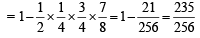Courses

# Test: MCQs (One or More Correct Option): Probability | JEE Advanced

## 19 Questions MCQ Test Maths 35 Years JEE Mains & Advance Past year Papers Class 12 | Test: MCQs (One or More Correct Option): Probability | JEE Advanced

Description
This mock test of Test: MCQs (One or More Correct Option): Probability | JEE Advanced for JEE helps you for every JEE entrance exam. This contains 19 Multiple Choice Questions for JEE Test: MCQs (One or More Correct Option): Probability | JEE Advanced (mcq) to study with solutions a complete question bank. The solved questions answers in this Test: MCQs (One or More Correct Option): Probability | JEE Advanced quiz give you a good mix of easy questions and tough questions. JEE students definitely take this Test: MCQs (One or More Correct Option): Probability | JEE Advanced exercise for a better result in the exam. You can find other Test: MCQs (One or More Correct Option): Probability | JEE Advanced extra questions, long questions & short questions for JEE on EduRev as well by searching above.
*Multiple options can be correct
QUESTION: 1

### If M and N are any two events, the probability that exactly one of them occurs is (1984 - 3 Marks)

Solution:

Given that M and N are any two events. To check the probability that exactly one of them occurs.
We check all the options one by one.
(a) P (M) + P (N) – 2 P (M ∩ N) = [P (M) + P (N) – P (M ∩ N)] – P( M ∩ N)
= P (M ∪ N) – P (M ∩ N)
⇒ Prob. that exactly one of M and N occurs.
(b) P (M) + P (N) – P (M ∩ N) = P (M ∪ N)
⇒ Prob. that at least one of M and N occurs.
(c) P (Mc) + P (Nc) – 2 P(Mc ∩ Nc) = 1– P (M) + 1 – P (N) – 2P (M ∪ N)c
= 2 – P (M)  – P (N) – 2 [1 – P (M ∪ N)]
= 2 – P(M) – P (N) – 2 + 2 P (M ∪ N)
= P (M ∪ N) +  P (M ∪ N) – P(M) – P (N)
= P (M ∪ N) – P (M ∩ N)
⇒ Prob. that exactly one of M and N occurs.
(d) P (M ∩ Nc) + P (M∩ N)
⇒ Prob that M occurs but not N or prob that M does not occur but N occurs.
⇒ Prob. that exactly one of M and N occurs.
Thus we can conclude that (a), (c) and (d) are the correct options.

*Multiple options can be correct
QUESTION: 2

### A student appears for tests I, II and III. The student is successful if he passes either in tests I and II or tests I and III. The probabilities of the student passing in tests I, II and III are p, q and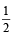respectively. If the probability that the student is successful is, then (1986 - 2 Marks)

Solution:

Let A, B, C be the events that the student passes test I, II, III respectively.
Then, ATQ ; P(A) = p; P (B) = q; P(C) =Now the student is successful if A and B happen or A and C happen or A, B and C happen.

ATQ,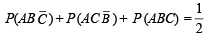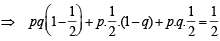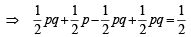⇒ p + pq = 1 ⇒ p (1 + q) = 1

which holds for p = 1 and q = 0.

*Multiple options can be correct
QUESTION: 3

### The probability that at least one of the events A and B occurs is 0.6. If A and B occur simultaneously with probability 0.2, then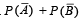is (1987 - 2 Marks) (Here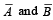are complements of A and B, respectively).

Solution:

Given that P (A ∪ B) = 0.6 ; P (A ∩ B) = 0.2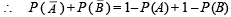= 2 – (P (A) + P (B)) = 2 –  [P (A ∪ B) + P (A ∩ B)].
= 2 – [0.6 + 0.2] = 2 – 0.8 = 1.2

*Multiple options can be correct
QUESTION: 4

For two given events A and B, P ( A ∩ B) (1988 - 2 Marks)

Solution:

We know that, P (A ∩ B) = P (A) + P (B) – P(A ∪ B) … (1)
Also P (A ∪ B) ≤ 1 ⇒ – P (A ∪ B) ≥ –1 … (2)
∴ P (A ∩ B) ≥ P (A) + P (B) –1 [Using (1) and (2)]
∴ (a) is true. Again P (A ∪ B) ≥ 0 ⇒ – P (A ∪ B) ≤ 0 … (3)
⇒ P (A ∩ B) ≤ P (A) + P (B) [Using (1) and (3)]
∴ (b) is also correct.
From (1) (c) is true and (d) is not correct.

*Multiple options can be correct
QUESTION: 5

If E and F are independent events such that 0 < P(E) <1 and 0 < P(F) < 1, then (1989 - 2 Marks)

Solution:

Since E and F are independent
∴ P (E ∩ F) = P (E) . P ( F) ...(1)
Now, P (E ∩ Fc) = P (E) – P (E ∩ F) = P (E) – P(E) P(F) [Using (1)]
= P (E) [1 – P (F)] = P (E) P (Fc)
∴ E and Fc are independent.
Again P (Ec ∩ Fc) = P (E ∪ F)c = 1 – P (E ∪ F) = 1– P (E) – P (F) + P (E ∩ F)
= 1 – P (E) – P (F) + P (E) P (F)
= ((1– P (E) (1 – P (F)) = P (Ec) P (Fc)
∴ Ec and Fc are independent.
Also P (E/ F) + P (Ec/F)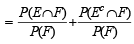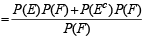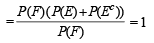*Multiple options can be correct
QUESTION: 6

For any two events A and B in a sample space (1991 -  2 Marks)

Solution:

For any two events A and B

(a)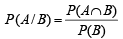Now we know P (A ∪ B) ≤ 1 P (A) + P (B) – P (A ∩ B) ≤ 1
⇒ P (A ∩ B) > P (A) + P (B) – 1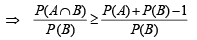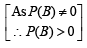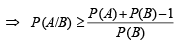∴ (a) is correct statement.

(b)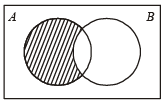From venn diagram we can clearly conclude that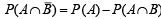∴ (b) is incorrect statement.

(c) P (A ∪ B) =P (A) + P (B) – P (A ∩ B)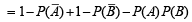[ ∵ A & B are independent events]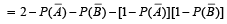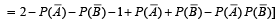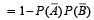∴ (c) is the correct statement.

(d) For disjoint events P (A ∪ B ) = P (A) + P (B)

∴ (d) is the incorrect statement.

*Multiple options can be correct
QUESTION: 7

E and F are two independent events. The probability that both E and F happen is 1/ 12 and the probability that neither E nor F happens is 1/2. Then, (1993 -  2 Marks)

Solution:

Let P (E) = x and P (F) = y

ATQ, P (E ∩ F) =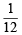As E and F are independent events

∴ P (E ∩ F) =  P (E) P (F)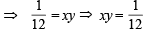… (1)

Also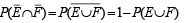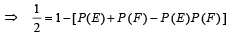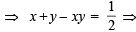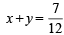… (2)

Solving (1) and (2) we get

either
x =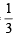and y =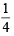or x =and y =∴ (a) and (d) are the correct options.

*Multiple options can be correct
QUESTION: 8

Let 0 < P(A) < 1, 0 < P(B) < 1 and P ( A ∪ B) = P(A) + P(B) – P(A)P(B) then (1995S)

Solution:

P (A ∪ B) ' = 1– P (A ∪ B)  = 1 – [P (A) + P (B) – P (A ∩ B)]
= 1 – P (A) – P (B) + P (A) P(B)
= P (A') P (B')
Also P (A ∪ B) = P (A) + P (B) – P (A) P (B)
⇒ P (A ∩ B) = P (A) + P (B)

∴ P (A/B) =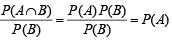*Multiple options can be correct
QUESTION: 9

If from each of the three boxes containing 3 white and 1 black, 2 white and 2 black, 1 white and 3 black balls, one ball is drawn at random, then the probability that 2 white and 1 black ball will be drawn is (1998 - 2 Marks)

Solution:

P (2 white and 1 black) = P (W1 W2 B3 or W1 B2 W3 or B1 W2 W3)
= P (W1 W2 B3) + P (W1 B2 W3) + P (B1 W2 W3)
= P (W1) P(W2) P (B3) + P (W1) P(B2) P (W3)
+ P (B1) P (W2) P (W3)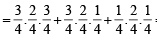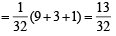*Multiple options can be correct
QUESTION: 10

If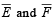are the complementary events of events E and F respectively and if 0 < P(F) < 1, then (1998 - 2 Marks)

Solution:

We have,

(a)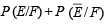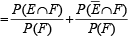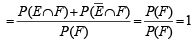∴ (a) holds.
Also

(b)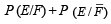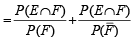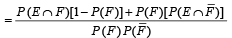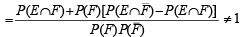∴ (b) does not hold. Similarly we can show that (c) does not hold but (d) holds.

*Multiple options can be correct
QUESTION: 11

There are four machines and it is known that exactly two of them are faulty. They are tested, one by one, in a random order till both the faulty machines are identified. Then the probability that only two tests are needed is(1998 - 2 Marks)

Solution:

The probability that only two tests are needed = (probability that the second machine tested is faulty given the first machine tested is faulty) =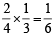*Multiple options can be correct
QUESTION: 12

If E and F are events with P(E) ≤ P(F) and P(E ∩ F) > 0, th en (1998 - 2 Marks)

Solution:

Given that P(E) ≤ P(F) and P (E ∩F )> 0. It doesn’t necessarily mean that E is the subset of F.
∴ The choices (a), (b), (c) do not hold in general.
Hence (d) is the right choice here.

*Multiple options can be correct
QUESTION: 13

A fair coin is  tossed repeatedly. If the tail appears on first four tosses, then the probability of the head appearing on the fifth toss equals (1998 - 2 Marks)

Solution:

Th e even t that th e fifth toss r esults in a h ead is independent of the event that the first four tosses result in tails.
∴ Probability of the required event = 1/2.

*Multiple options can be correct
QUESTION: 14

Seven white balls and three black balls are randomly placed in a row. The probability that no two black balls are placed adjacently equals (1998 - 2 Marks)

Solution:

The no. of ways of placing 3 black balls without any restrition is 10C3. Now the no. of ways in which no two black balls put together is equal to the no of ways of choosing 3 places marked out of eight places. – W– W – W – W – W – W – W –
This can be done is 8C3 ways. Thus, probability of the

required event =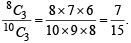∴ (b) is the correct option.

*Multiple options can be correct
QUESTION: 15

The probabilities that a student passes in Mathematics, Physics and Chemistry are m, p and c, respectively. Of  these subjects, the student has a 75% chance of passing in at least one, a 50% chance of passing in at least two, and a 40% chance of passing in exactly two. Which of the following relations are true? (1999 - 3 Marks)

Solution:

According to the problem, m + p + c – mp – mc – pc + mpc = 3/4 …(1)
mp (1– c) + mc (1– p) + pc (1– m) = 2/5 or mp +   mc + pc – 3mpc = 2/5 … (2)
Also mp  + pc + mc – 2mpc = 1/2 … (3)

(2) and (3) ⇒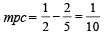∴ mp + mc + pc =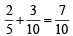∴ m + p + c =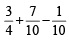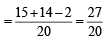*Multiple options can be correct
QUESTION: 16

Let E and F be two  independent events. The probability that exactly one of them occurs is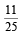and the probability of none of them occurring is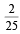. If P(T) denotes the probability of occurrence of the event T, then (2011)

Solution:

Q E and F are independent events

∴ P( E ∩F) = P(E). P(F) ...(1)

Given that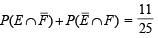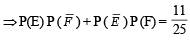⇒ P(E) ( 1 – P (F)) + (1 – P (E)) P (F) =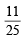⇒ P (E) – P (E) P (F) + P (F) – P(E) P (F) =⇒ P (E) + P (F) – 2 P(E). P(F) =...(2)

and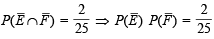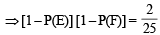⇒ 1 – P(E) – P(F) + P(E) P(F) =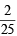...(3)

Adding equation (2) and (3) we get

1 – P(E) P(F) =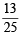or    P(E) P(F) =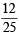...(4)

Using the result in equation (2) we get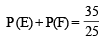...(5)

Solving (4) and (5) we get

P(E) =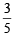and P(F) =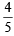or P(E) =and P(F)  =∴ (a) and (d) are the correct options.

*Multiple options can be correct
QUESTION: 17

A ship is fitted with three engines E1 , E2  and E3 . The engines function independently of each other with respective  probabilities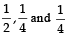For  the  ship  to  be operational at  least  two  of  its  engines  must function. Let X denote the event that the ship is operational and let X1 , X2  and X3  denote respectively the events that the engines E1, E2 and E3  are functioning. Which of the following is(are) true ? (2012)

Solution:

We have P(X1) =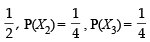P(X) = P(at least 2 engines are functioning)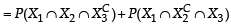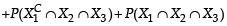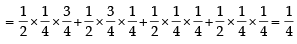(a)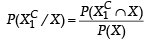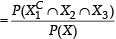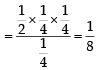(b) P [Exactly two engines are functioning /X]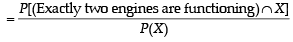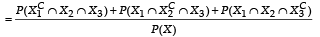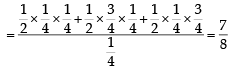(c)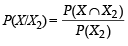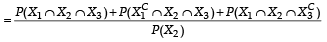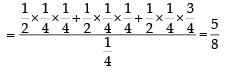(d)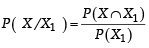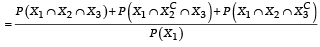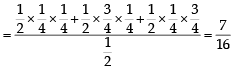*Multiple options can be correct
QUESTION: 18

Let X and Y be two even ts such that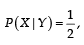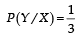and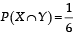Which of the following is (are) correct ? (2012)

Solution:

We know P(X/Y) =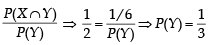Similarly,  P(Y/X) =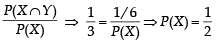∴ P(X ∪ Y) = P(X) + P(Y) – P(X ∩ Y)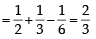Also P(X ∩ Y) = P(X)P(Y) ⇒ X and Y are independent events.
∴ XC and Y are also independent events.

∴ P(XC ∩ Y) = P(XC) × P(Y)  =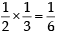*Multiple options can be correct
QUESTION: 19

Four persons independently solve a certain problem correctly with probabilities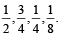Then the probability that theproblem is solved correctly by at least one of them is (JEE Adv. 2013)

Solution:

P (atleast one of them solves the problem) = 1 – P (none of them solves it)